Analysis WebNotes
arrow_back arrow_forward

# Class Contents

## Subsequences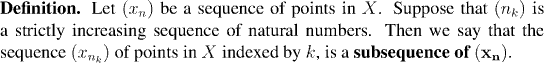In the following examples, we take the sequence: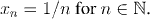Example: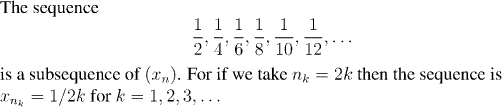Example: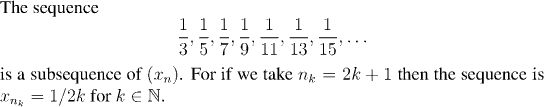Example: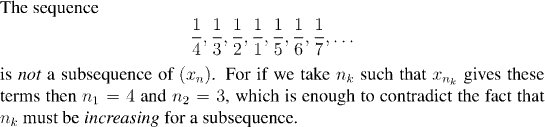Example: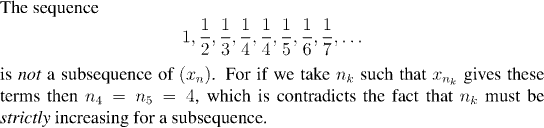Example: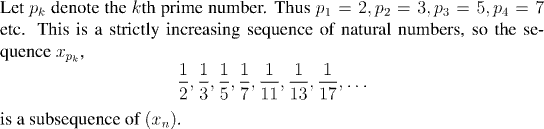One important reason subsequences are useful is because very often even when a sequence does not converge itself, it does have subsequences which converge.

Example: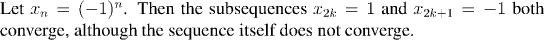On the other hand, if a sequence does converge then all its subsequences also converge, and to the same thing.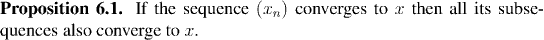Remark:

Although it seems a pretty unsurprising result, this last proposition does give us a useful criterion for establishing that a given sequence does not converge. All one has to do is to identify two subsequences which converge to different limits. Since we have far more results at our disposal which do prove convergence than we have which disprove it, this is oftentimes much easier.

Example: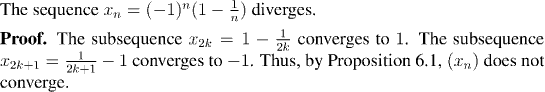The following theorem shows that, at least in the real numbers, many sequences have convergent subsequences. So this behavior is very common indeed.Remark:

This proof of the Bolzano-Weierstrass theorem uses ideas based on Ramsey's Theorem from combinatorics. Indeed the key step of the proof, showing that every sequence of real numbers has a monotonic subsequence can be proved using Ramsey's Theorem.

We can use Ramsey's theorem to prove again that every sequence of real numbers has a monotonic subsequence: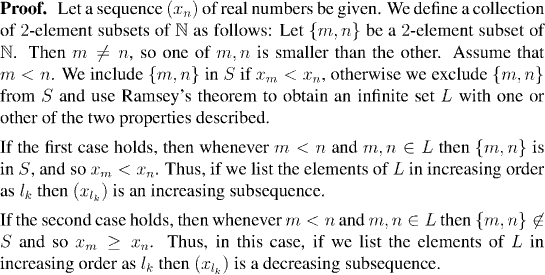Following on the Bolzano-Weierstrass Theorem, we can use obtain a useful property of closed and bounded subsets of the real numbers: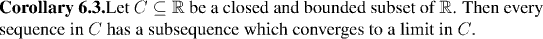This property is studied in more detail in a future section.